# Time Series Analysis for Alternative Data

As Chief Data Scientist at Adaptive Management, my goal is to make data simple for our clients. Alternative data sources (such as credit card receipts, footfall traffic, satellite imagery, etc.) are non-traditional data sources that offer a scalable, quantified measure of performance to support theses on a current or future investment. Time series analysis is essential for estimating the values of fragmented, missing, or future data sources — these situations are common in modeling key performance indicators.

While time series analysis may appear as a black box, it is our goal to bring some transparency to the algorithms that are available in DataMonster™. We highlight the integral steps of time series analysis but avoid going into the technical details of parameter optimization.

# Time Series Analysis

Time series analysis aims to understand the structures and patterns in time series data. These patterns are useful to describe trends and seasonal properties of the data as well as to forecast future values of the data. Simplicity is prefered over complexity in the analysis for the following reasons:

• Reduce the risk of over-fitting. A model has a number of parameters with optimized values over a subset (training set) of the time series — the remainder of the time series becomes the validation set. Over-fitting occurs when the model accurately describes the training set but fails to accurately describe the validation set. For time series, the validation set is often a hold out period. If the model fails to accurately describe the training set then the model may be too simple or the training set may be too small.
• Minimize the computational cost of the analysis. When computing forecasts for a large number of time series, computation costs and time are critical components. It is common to train neural networks for weeks or months — this may be too long to be applicable to the problem. When working with alternative data sets, we often analyze a large number of time series. This number can be further increased by subsampling the time series on another data field. In all cases, you should expect an increase in accuracy for extra computational cost.
• Tractable error rates. Error rates in complex models are often computed using Monte-Carlo simulations. Statistically properties of some simpler models can be computed analytically (as opposed to numerically), resulting in easier to compute error bounds and lessening the data requirements. More complex models may require significantly more computation and data to provide reasonable confidence intervals for associated forecasts.
• Interpretability. Some complex models are essentially black boxes with parameters that are difficult to relate to real world phenomena. The simpler model has to be more judicious in its choice of parameters. To support a thesis, it is helpful to translate the value of a parameter to a real world metric. Insights from subject matter experts may constrain the parameters, increasing the relevance of the model.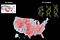Simplicity and Complexity of Models. If one is attempting to forecast a national election result then state-wide fidelity may be too simplistic for accurate predictions. In the absurd extreme, too much information (e.g. genetics) would result in an overly complicated model. Finding the correct number of parameters (perhaps county-wide resolution) is the challenge of creating a good model.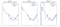Underfitting and Overfitting Model. The line does not fully describe the structure of the true function. The degree 15 polynomial describes the deviations more than the underlying true function.

The right amount of complexity/parameters in a model balances extracting structures and of patterns from the data with overfitting. The goal is have the deviations from the model be well-modeled by a stochastic process. For example, the flip of a coin is deterministic with complex enough physical model that includes aerodynamics and rigid body mechanics. For simplicity, it is usually sufficient to model the coin flip as a Bernouli random process, where the probability of heads and of tails are included in a model but all of the physics is absorbed into the noise model.

# The Path to Stationarity

For our forecasts, we structure the time series model to include the general trend of growth, annual (and weekly) seasonal components, and influence from holidays. Our forecast is powerful if the residuals of the model, the components not included in the model, have nice statistical properties. For time series analysis, the goal is to have the statistical properties of the excluded components be well modeled by a stationary process, meaning the the statistical properties do not change over time.

We will describe the transformations of the time series that define our model; each additional transformation brings the residuals closer to the desired stationary state.

## Trend or Linear Growth

Trend is the long term growth of a time series without any seasonal or holiday components. We compute a rolling mean over a year to model the trend of a time series. To simplify the rolling mean, we compute a piecewise-linear model with optimal choice of change points. The distributions of the times of the change points and of the linear coefficients of the linear models allows us to forecast the trend into the future. More complex algorithms can be employed to describe the trend at an increased computational cost.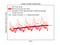Extracting Trend. The linear growth is extracted from a moving average. The noise model from the forecast is derived from the distribution of change points in the piecewise-linear approximation. The validity of the linear extrapolation may be limited in time.Removal of Trend. The red points are the original time series and the blue points are after the removal of the linear trend removed. It is visually evident that the residuals are not stationary.

## Seasonality

Seasonality is the periodic component to a time series. For many data sources, the natural periods are yearly or weekly. To extract the seasonal components, we filter the top Fourier modes (a calculation that sorts the amplitudes of different frequencies of the time series).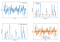Filtration of Time Series to Find Seasonality. The largest amplitude frequencies are used to forecast seasonality in the model. The filtration process starts in the time domain (upper left). Next we transform to the frequency domain (upper right). We remove weaker frequencies (lower left). The remaining frequencies (the ones that pass through our filter) are used to model seasonality in the forecast (lower right).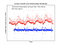Seasonality Filter. The red point are the residuals of the time series after removals of the linear growth and seasonal component. It is close to appearing stationary but there are still outliers that may be accounted for by a holiday analysis.

## Holidays

Holidays are points in the series where we anticipate large deviations from the model. Sometimes these holidays are on a fixed date such as Christmas and sometimes the holidays move around such as Thanksgiving. We generalize holidays to describe days that are also near typical holidays such as Black Friday. We extract the average value of the time series on the holidays from the signal.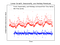Filter out Holiday Deviations. The red points are the residuals after extracting the linear growth, seasonality, and holiday components. There are still some large deviations that are likely a result of unknown holiday-like events or noise in the data. One can balance overfitting with removal of the larger deviations. But for our purposes, we treat the blue time series as stationary.

This decomposition is not perfect. There may be holiday-like events, e.g. large deviations from the model, that we do not include, such as an Amazon Prime day. The goal is to arrive at a stationary distribution after extracting trend, seasonality, and holidays. By stationary, we mean that the remainder is well modeled as a random process with constant mean and constant variance.

## Forecasting

Putting the components together, we can forecast the time series into the future. Assuming the noise in each component is normally distributed and independent, the variance of the forecast is the sum of the variances of each component:

Each of these components along the path to stationarity are part of the complete model.

The complexity ignored by the model is modeled by the Gaussian noise. After extracting the trend, seasonality, and holiday components, we can compute the statistical parameters of the residual noise.

To fit the model to training data in the time series, we optimize the choice of parameters to minimize the mean squared error (MSE) between the model predictions and the training data.

## Test for Stationarity

There are multiple ways to determine if the model is sufficiently descriptive for forecasting. The primary test is a backtest that holds out a subset of the data and uses the model to forecast the held out values.

Even without backtesting, we can estimate how good the model is by the statistical properties of the residuals. The model is well-suited for forecasting when the residuals are described by a stationary process. There are several tests (e.g. Dickey-Fuller or Neyman-Pearson) to determine if this is indeed the case. The gist of the tests is to present two models — one non-stationary and one stationary — and determine which model the data fit better.

# Alternative forecasts and ARIMA

## Bayesian Inference

Having prior assumptions on the distributions of the parameters of the model enables us to employ Bayesian inference to improve the optimization. While fitting the model, we jointly optimize the trend, seasonality, and holiday parameters. However, we obtain similar quality results with a fraction of the computational cost by judiciously prescribing the location of changepoints in the trend and subsequently decomposing the optimization by component.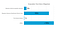Order of Magnitude of More Complex Time Series Analysis Methods. The accuracy levels of other time series analysis techniques did not improve accuracy of forecasts. However, the computational time is significantly cheaper for the presented method (labeled Time Series Analysis). Furthermore, our analysis is parallelizable across GPUs and have very modest memory requirements.

## ARIMA

One powerful collection of algorithms for computing forecasts are grouped together and called ARIMA (AutoRegression, Integration, and Moving Average). While ARIMA algorithms have some potential advantages, many of the techniques one would use to get to stationarity for ARIMA are precisely what we compute in our decomposition of time series into trend, seasonality, and holiday components. Several reasons why we choose not to employ ARIMA at scale are the following:

• typically more expensive (with respect to the number of calculations and time to compute) than the decomposition described above especially for yearly seasonality on daily data,
• have hyperparameters that need to be optimized,
• typically good for short-term forecasts but start to drift after that,
• computation of confidence intervals and uncertainty is more difficult,
• lacks the ability to understand the parameters/decomposition.

A more technical discussion that compares ARIMA to a Bayesian approach can be found here.

# Summary

The strength of our model is the ability parallelize its computation across a large number of time series since each step is a relatively simple computation. However, every model is limited by the training data. If the training data is not representative of future values, then the model will be lacking. The variance in the forecast is computed under the assumptions that the patterns and trends extracted from training data are similar to future data.

Time Series Analysis is not a black box. The goal is to extract enough useful structure from your data to be predictive. After the extraction/decomposition of the structures, the remainder is often well-modeled by a common statistical model. Forecasts and confidences intervals are based on the statistical model and the extracted structures.

Written by

Written by

## More From Medium

Welcome to a place where words matter. On Medium, smart voices and original ideas take center stage - with no ads in sight. Watch
Follow all the topics you care about, and we’ll deliver the best stories for you to your homepage and inbox. Explore
Get unlimited access to the best stories on Medium — and support writers while you’re at it. Just \$5/month. Upgrade